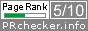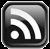# LEARN MS-ACCESS TIPS AND TRICKS

Learn Microsoft Access Advanced Programming Techniques, Tips and Tricks.

### Introduction.

Normally we round numbers in calculations when the fractional part is 0.5 or more to 1 (next highest integer) or truncates it all together. But, there are times that this fractional part itself to be rounded to a certain level so that it can be used for disbursement of money, like wages.

For example, in Currencies when the value ends up in 15 cents, 30 cents they should be rounded to 25 cents, 50 cents respectively for determining the requirement of correct number and denominations of Coins for disbursement. If we decide we need only 50 Cents Coins then a value of 25 Cents or more should be rounded to 50 Cents and less than 25 Cents to zero.

So, we need a function that can accept a value as precision and find multiples of that value and when the remainder value is half or more of the precision value it should be rounded to the next level. Not necessary that it should be a fractional value it can be any value as precision.

In MS-Access we have Round() Function that will only round the Double Precision numbers into the required number of Decimal Places or to the next integer level applying the normal rules that we are already familiar with. There is a Worksheet Function MRound() in MS-Excel that can do these kinds of calculations but found nothing like that in MS-Access. We cannot go to Excel when we want this in Access.

### The MRound Function

We will write an MRound() Function in Access with the same name.

So here it is:

```Public Function MRound(ByVal Number As Double, ByVal Precision As Double) As Double
Dim Y As Double

On Error GoTo MRound_Err

Y = Int(Number / Precision) * Precision
MRound = IIf(((Number - Y) / Precision * 100 + 0.1) >= 50, Y + Precision, Y)

MRound_Exit:
Exit Function

MRound_Err:
MsgBox Err.Description, , "MRound()"
MRound = 0
Resume MRound_Exit
End Function
```

### Save the Function and do Test Runs.

Copy and paste the above Code into a Global Module of your Database and save the Module.

Open the Debug Window (Ctrl+G) to try it out directly.

Sample Runs:

? Mround(123.3,.2)

Result: 123.4

? Mround(123.24,.5)

Result: 123

? Mround(123.25,.5)

Result: 123.5

? Mround(123.74,.5)

Result: 123.5

? Mround(123.75,.5)

Result: 124

? Mround(10,3)

Result: 9

? Mround(11,3)

Result: 12

If you would like to share this Function across your Other MS-Access Databases then create a Library Database with the Function in it and set a Reference to the Library Database through Tools- ->References in VBA Window.

Share:

1.Nice function, although I wish all Excel formulas were inherently available in Access. Is there a similar function/formula that will do the same with ROUNDUP. I’d like to use it for retail pricing where Mround(123.24,.5) would result in: 123.50.

2.Thanks for the simplified formula. My work demands the use of this function. Thanks to you that I can simply use this to calculate the days quickly without wasting time.

Comments subject to moderation before publishing.

## TranslateSubscribe in a readerYour email address:

Delivered by FeedBurner